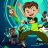# Standard deviation from linear regression curve?

A

#### arod49

##### New member
Can someone make the linear regression curve indicator have standard deviation?#### wtf_dude

##### Active member
Here ya go. Not sure what you wanted it to look like so it just looks like the Bollingers

Code:
``````#LinearRegwithSTDevBands
#mods by WTF_Dude

input displace = 0;
input length = 9;
input price = close;

plot LinReg = Inertia(price[-displace], length);
LinReg.SetDefaultColor(GetColor(1));

input SD_length = 14;
input Num_Dev_Dn = -2.0;
input Num_Dev_up = 2.0;

def sDev = StDev(data = LinReg[-displace], length = SD_length);

plot LowerBand = LinReg + Num_Dev_Dn * sDev;
plot UpperBand = LinReg + Num_Dev_up * sDev;

LowerBand.SetDefaultColor(GetColor(0));
#LowerBand.setstyle(Curve.Long_DASH);
LinReg.SetDefaultColor(GetColor(1));
#LinReg.setstyle(Curve.MEDIUM_DASH);
UpperBand.SetDefaultColor(GetColor(5));
#UpperBand.setstyle(Curve.Long_DASH);``````
. I set standard deviation to the regular 14 length that the TD indicator uses by default.

•BenTen
A

#### arod49

##### New member
thank you very much sir

•wtf_dude#### wtf_dude

##### Active member
thank you very much sir
no problem. let me know if you get any good results using it in a system

•arod49Auto Volatility Standard Deviation Levels for ThinkorSwim Custom 19